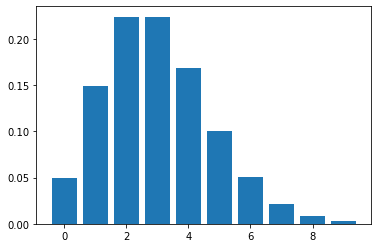# Day02 典型統計應用在社群媒體分析(Classical statistics applied to social data) part 2

<續前>

• 來自消防局的一小時間的tweet數量 $\longrightarrow$ 大量的 N
• 使用者位置 = "Boulder, Colorado, United States"的機率 $\longrightarrow$ 少量的 $p$

$P(k;\nu) = \frac{\nu^k e^{-\nu}}{k!}$

nu = 3 # Poisson mean...the most likely value
n = nu*3 # this simply sets a range; it is not a parameter of the distribution

k = scipy.linspace(0,n,n+1) # create bins
pmf = scipy.stats.poisson.pmf(k,nu)

plt.bar(k,pmf)• 這個分布是一個離散變數函數
• 這個分布有一個連續參數(continuous parameter)
• 對於少量 v 這個分布是不對稱的
• 對於大量 v 這個分布有變成對稱

## 假設檢定(Hypothesis Tests) 和 p-值(p-values)

### 假設類型

• 使用者位置 = "Boulder, Colorado, United States"的 tweets 的比率是帕松分布(Poisson distribution)，且平均值是 4.5/小時
• 使用者位置 = "Boulder, Colorado, United States"的 tweets 的比率是帕松分布(Poisson distribution)，且平均值是 4.5/10k tweets
• 在過去1小時 提到 @ 多於1000次的 Twitter 使用者人數是常態分佈且平均值是 301，變異數是 200。